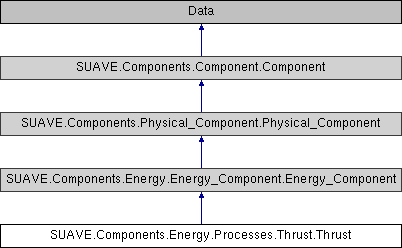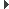SUAVE  2.5.2 An Aerospace Vehicle Environment for Designing Future AircraftSUAVE.Components.Energy.Processes.Thrust.Thrust Class Reference
Inheritance diagram for SUAVE.Components.Energy.Processes.Thrust.Thrust:## Public Member Functions

def __defaults__ (self)

def compute (self, conditions)

def compute_stream_thrust (self, conditions)

def size (self, conditions)

def size_stream_thrust (self, conditions)

## Public Attributes

tag

bypass_ratio

compressor_nondimensional_massflow

reference_temperature

reference_pressure

number_of_engines

design_thrust

mass_flow_rate_designPublic Attributes inherited from SUAVE.Components.Energy.Energy_Component.Energy_Component
inputs

outputsPublic Attributes inherited from SUAVE.Components.Physical_Component.Physical_Component
tag

mass_properties

origin

symmetricPublic Attributes inherited from SUAVE.Components.Component.Component
tag

origin

generative_design_max_per_vehicle

generative_design_characteristics

generative_design_special_parent

## Detailed Description

```A class that handles computation of thrust and other outputs for a gas turbine engine.

Assumptions:
Perfect gas

Source:
https://web.stanford.edu/~cantwell/AA283_Course_Material/AA283_Course_Notes/
```

## ◆ __defaults__()

 def SUAVE.Components.Energy.Processes.Thrust.Thrust.__defaults__ ( self )
```This sets the default value.

Assumptions:
None

Source:
N/A

Inputs:
None

Outputs:
None

Properties Used:
N/A
```

Reimplemented from SUAVE.Components.Energy.Energy_Component.Energy_Component.

## ◆ compute()

 def SUAVE.Components.Energy.Processes.Thrust.Thrust.compute ( self, conditions )
```Computes thrust and other properties as below.

Assumptions:
Perfect gas

Source:
https://web.stanford.edu/~cantwell/AA283_Course_Material/AA283_Course_Notes/

Inputs:
conditions.freestream.
isentropic_expansion_factor        [-] (gamma)
specific_heat_at_constant_pressure [J/(kg K)]
velocity                           [m/s]
speed_of_sound                     [m/s]
mach_number                        [-]
pressure                           [Pa]
gravity                            [m/s^2]
conditions.throttle                  [-] (.1 is 10%)
self.inputs.
fuel_to_air_ratio                  [-]
total_temperature_reference        [K]
total_pressure_reference           [Pa]
core_nozzle.
velocity                         [m/s]
static_pressure                  [Pa]
area_ratio                       [-]
fan_nozzle.
velocity                         [m/s]
static_pressure                  [Pa]
area_ratio                       [-]
number_of_engines                  [-]
bypass_ratio                       [-]
flow_through_core                  [-] percentage of total flow (.1 is 10%)
flow_through_fan                   [-] percentage of total flow (.1 is 10%)

Outputs:
self.outputs.
thrust                             [N]
thrust_specific_fuel_consumption   [N/N-s]
non_dimensional_thrust             [-]
core_mass_flow_rate                [kg/s]
fuel_flow_rate                     [kg/s]
power                              [W]
Specific Impulse                   [s]

Properties Used:
self.
reference_temperature              [K]
reference_pressure                 [Pa]
compressor_nondimensional_massflow [-]
```

## ◆ compute_stream_thrust()

 def SUAVE.Components.Energy.Processes.Thrust.Thrust.compute_stream_thrust ( self, conditions )
```Computes thrust and other properties as below.

Assumptions:

Source:
Heiser, William H., Pratt, D. T., Daley, D. H., and Unmeel, B. M.,
"Hypersonic Airbreathing Propulsion", 1994
Chapter 4 - pgs. 175-180

Inputs:
conditions.freestream.
isentropic_expansion_factor        [-]
specific_heat_at_constant_pressure [J/(kg K)]
velocity                           [m/s]
speed_of_sound                     [m/s]
mach_number                        [-]
pressure                           [Pa]
gravity                            [m/s^2]
conditions.throttle                  [-] (.1 is 10%)

self.inputs.
fuel_to_air_ratio                  [-]
total_temperature_reference        [K]
total_pressure_reference           [Pa]

core_nozzle.
velocity                         [m/s]
static_pressure                  [Pa]
area_ratio                       [-]

fan_nozzle.
velocity                         [m/s]
static_pressure                  [Pa]
area_ratio                       [-]
number_of_engines                [-]
bypass_ratio                     [-]
flow_through_core                [-] percentage of total flow (.1 is 10%)
flow_through_fan                 [-] percentage of total flow (.1 is 10%)

Outputs:
self.outputs.
thrust                             [N]
thrust_specific_fuel_consumption   [N/N-s]
non_dimensional_thrust             [-]
core_mass_flow_rate                [kg/s]
fuel_flow_rate                     [kg/s]
power                              [W]

Properties Used:
self.
reference_temperature              [K]
reference_pressure                 [Pa]
compressor_nondimensional_massflow [-]
```

## ◆ size()

 def SUAVE.Components.Energy.Processes.Thrust.Thrust.size ( self, conditions )
```Sizes the core flow for the design condition.

Assumptions:
Perfect gas

Source:
https://web.stanford.edu/~cantwell/AA283_Course_Material/AA283_Course_Notes/

Inputs:
conditions.freestream.speed_of_sound [m/s] (conditions is also passed to self.compute(..))
self.inputs.
bypass_ratio                       [-]
total_temperature_reference        [K]
total_pressure_reference           [Pa]
number_of_engines                  [-]

Outputs:
self.outputs.non_dimensional_thrust  [-]

Properties Used:
self.
reference_temperature              [K]
reference_pressure                 [Pa]
total_design                       [N] - Design thrust
```

## ◆ size_stream_thrust()

 def SUAVE.Components.Energy.Processes.Thrust.Thrust.size_stream_thrust ( self, conditions )
```Sizes the core flow for the design condition.

Assumptions:
Perfect gas

Source:
Heiser, William H., Pratt, D. T., Daley, D. H., and Unmeel, B. M.,
"Hypersonic Airbreathing Propulsion", 1994
Chapter 4 - pgs. 175-180

Inputs:
conditions.freestream.speed_of_sound [m/s] (conditions is also passed to self.compute(..))
self.inputs.
bypass_ratio                       [-]
total_temperature_reference        [K]
total_pressure_reference           [Pa]
number_of_engines                  [-]

Outputs:
self.outputs.non_dimensional_thrust  [-]

Properties Used:
self.
reference_temperature              [K]
reference_pressure                 [Pa]
total_design                       [N] - Design thrust ```

The documentation for this class was generated from the following file:
• /Users/emiliobotero/Dropbox/SUAVE/SUAVE/trunk/SUAVE/Components/Energy/Processes/Thrust.py